Officially, Home Plate doesn’t exist.

The white shape above which bat meets ball is a 17” square minus the two corners that would lie in foul territory.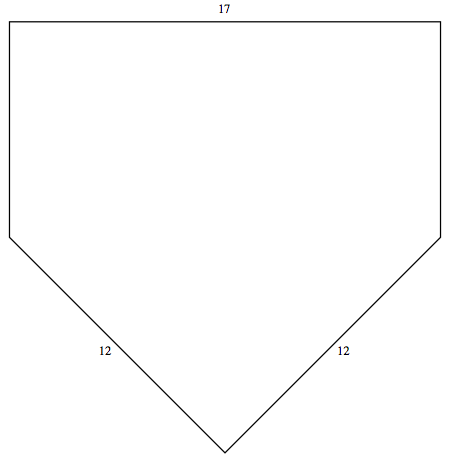The official Major League and Little League rule books require the two “slanty” sides to be 12” long  and meet at a right angle at the rear corner toward the catcher.  This is where the foul lines meet.  The left and right sides of Home Plate must poke into fair territory by half the width of the plate, which is 8½” (17” divided by 2).

There is no such shape!

Those slanty sides have to be slightly longer than 12”!  Either that or the corners can’t be exactly right angles.  This is explained at the website http://mathworld.wolfram.com/HomePlate.html, but it requires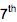grade math to understand.

Here’s an easier way.  If there were such a shape, the big right triangle at the bottom (which is half of a square cut diagonally)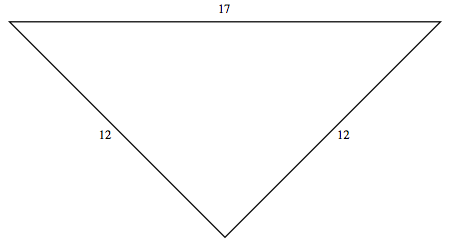would be 12” by 12” by 17”, the same width as the whole plate (the so-called Strike Zone).  Cut this triangle out of paper.  No, wait, that’s impossible.  Carefully cut a diagonal half square from paper. It can be smaller, say 12 by 12 by (roughly) 17 centimeters, or any size.  Only the shape matters.  It will be a scale model, cut from a square 12 “pretend inches” on a side, and supposedly 17 “pretend inches” across.  Now fold the lower left edge onto the top edge as shown, dividing the 45° angle in half: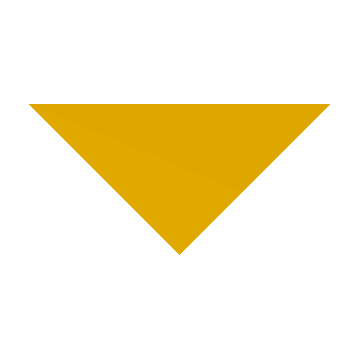It makes a baby diagonal half-square on the right!  If the original sides were 12 and 17,
the new sides are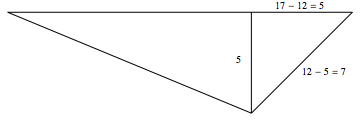The 5 is what’s left sticking out when we fold the 12 up onto the 17.  The vertical side is also 5 because it’s the other side of a half-square, leaving for its diagonal side 12 - 5 = 7 of the original “slanty” side.  So if 12, 12, 17 is a diagonal halfsquare, we can fold it and make a 5, 5, 7 one.  And we can do it again!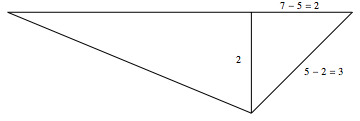So 2,2,3 must also be a diagonal half-square.  And we can keep going!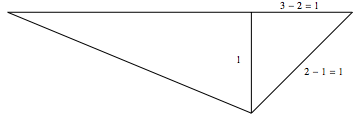So 1,1,1, which is an equilateral triangle, must also be a diagonal half-square.  That sounds a little fishy, but if it really is a half-square, we can keep going:WHAT??  A 0,0,1 triangle?  If the sides are 0 long, how can we see them?  This is some triangle.  It’s shorter to go the long way around, 0 + 0, than it is to go 1 straight along the diagonal!  Bad news, sports fans, this is not a triangle.

We must have blown it someplace.  But all we did was some subtractions, and those are OK.  The only possible place we messed up was to believe that the original triangle 12, 12, 17, was a diagonal half-square.

That’s why the baseball rulebooks are wrong.

Let’s be nice and tell them the numbers they should have used.  Whole inches are probably too big.  Maybe we should use millimeters, so the correct size will be something like 305 by 305 by 432 millimeters.  But wait a minute.  If that’s really the right shape, then we can do the same folding trick and make a smaller half-square with whole numbers, because we only subtract.  And we can keep it up until we get one of those ridiculous triangles with nonexistent sides!

What’s going on?  As soon as we say two numbers, the paper fold says two smaller ones.  We can’t win.  Where did we mess up this time?  The only possible mistake we made was to try to describe Home Plate with whole numbers.  It can’t be done.